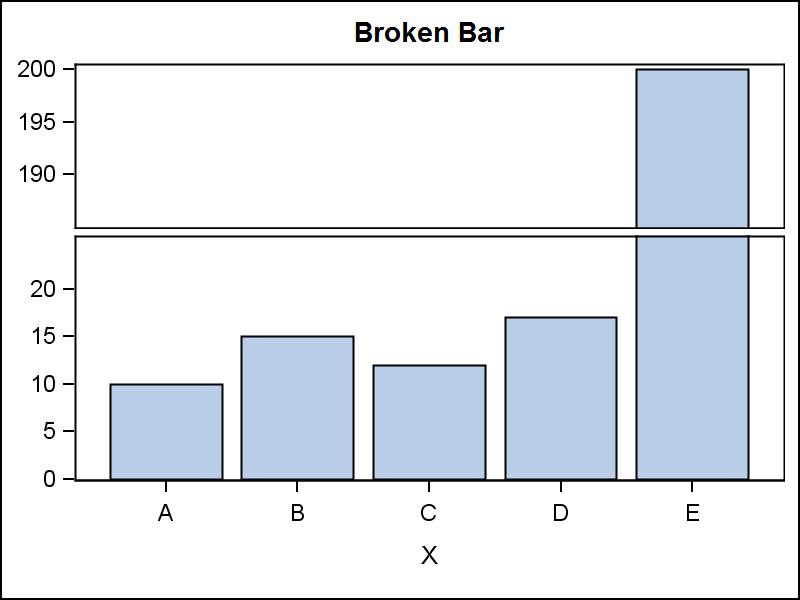## histogram with break in y axis

hi,

I need to compare the histogram of a discrete but quantitative variable (that has about 30 values with increment of one) between two groups. the last value of the x-variable has a mass of frequency much much higher that the others. Since I would like to show all the values on the x-axis, maybe a discontinuous Y axis would be the right way.

In alternative I can also use a line graph, but for the same reason I would need a break on the Y axis.

Is there a way to create such a graph possibly with an sg graph solution?

I'm running 9.2

Any help is greatly appreciated

5 REPLIES 5

## Re: histogram with break in y axis

no hints?

isn't it possible with gtl? I would appreciate if someone could suggest a code for a problem like this.

anyway thank you all very much

## Re: histogram with break in y axis

The way statisticians handle this issue is to use a log-scale axis for Y. This is seen as being more "honest" than to break the axis.

A second approach is to alter the data so that the tall bar is not as tall, and then put an arrow over it (pointing up) with the true value.Jay54
Meteorite | Level 14

## Re: histogram with break in y axis

Rick's idea is the easiest.

Now, you could use a GTL Lattice Layout with a two cell stack.  Pre summarize (or bin) the data, and use a HistogramParm or a BarChart in each cell.  Make upper cell shorter.  Set the Y axis Viewmin and Viewmax to see the ranges you need.  Here is example.  Let me know if this work for you?data TallBar;
input X \$ Y;
datalines;
A  10
B  15
C  12
D  17
E  200
;
run;
proc print;run;

proc template;
define statgraph tall_bar;
begingraph;
entrytitle 'Broken Bar';
layout lattice / rows=2 columndatarange=union rowweights=(0.4 0.6) rowgutter=5;

columnaxes;
columnaxis;
endcolumnaxes;

layout overlay / yaxisopts=(display=(ticks tickvalues)
offsetmin=0 linearopts=(viewmin=185 tickvaluelist=(190 195 200)));
barchart x=x y=y;
endlayout;
layout overlay / yaxisopts=(display=(ticks tickvalues)
offsetmin=0 linearopts=(viewmax=25 tickvaluelist=(0 5 10 15 20)));
barchart x=x y=y;
endlayout;
endlayout;
endgraph;
end;
run;

ods graphics / reset width=4in height=3in imagename='Tall_bar';
proc sgrender data=TallBar template=tall_bar;
run;

## Re: histogram with break in y axis

wow, the example above seems what I was ooking for. I'll try to apply to my data and adapt to the group comparison

the idea of altering the taller bar is also very nice and looks quite simple. thank you very much to both.Jay54
Meteorite | Level 14

## Re: histogram with break in y axis

See another idea (SAS 9.3) in blog article on Broken y-Axis.

Discussion stats
• 5 replies
• 1857 views
• 1 like
• 3 in conversation